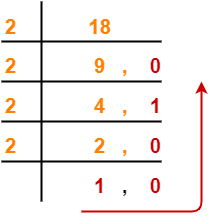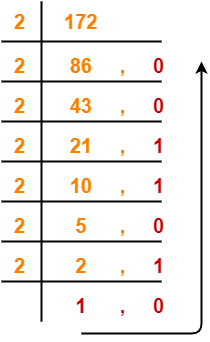# Decimal to Binary Conversion | Base 10 to base 2

## Number System Conversions-

Before you go through this article, make sure that you have gone through the previous article on Basics of Number System.

In number system,

• It is very important to have a good knowledge of how to convert numbers from one base to another base.
• Here, we will learn how to convert any given number from base 10 to base 2.## Decimal to Binary Conversion-

A given number can be converted from base 10 to any other base using division method and multiplication method.

 Learn how much goods and services should cost from painting a car or reupholstering a car windshield to hiring wedding planner or a cook at The Pricer.

Following two cases are possible-

### Case-01: For Numbers Carrying No Fractional Part-

• Division Method is used to convert such numbers from base 10 to another base.
• The division is performed with the required base.

### Steps To Convert From Base 10 to Base 2-

• Divide the given number (in base 10) with 2 until the result finally left is less than 2.
• Traverse the remainders from bottom to top to get the required number in base 2.

### Case-02: For Numbers Carrying A Fractional Part-

To convert such numbers from base 10 to another base, real part and fractional part are treated separately.

### For Real Part-

The steps involved in converting the real part from base 10 to another base are same as above.

### For Fractional Part-

• Multiplication Method is used to convert fractional part from base 10 to another base.
• The multiplication is performed with the required base.

### Steps To Convert From Base 10 To Base 2-

• Multiply the given fraction (in base 10) with 2.
• Write the real part and fractional part of the result so obtained separately.
• Multiply the fractional part with 2.
• Write the real part and fractional part of the result so obtained separately.
• Repeat this procedure until the fractional part remains 0.
• If fractional part does not terminate to 0, find the result up to as many places as required.

Required Number in Base 2

= Series of real part of multiplication results obtained in the above steps from top to bottom

Also Read- Conversion to Base 10

## Problems-

Convert the following numbers from base 10 to base 2-

1. (18)10
2. (18.625)10
3. (172)10
4. (172.878)10

## Solution-

### 1. (18)10

#### (18)10 → ( ? )2

Using division method, we have-From here, (18)10 = (10010)2

### 2. (18.625)10

#### (18.625)10 → ( ? )2

Here, we treat the real part and fractional part separately-

### For Real Part-

• The real part is (18)10
• We convert the real part from base 10 to base 2 using division method same as above.

So, (18)10 = (10010)2

### For Fractional Part-

• The fractional part is (0.625)10
• We convert the fractional part from base 10 to base 2 using multiplication method.

Using multiplication method, we have-

 Real part Fractional Part 0.625 x 2 1 0.25 0.25 x 2 0 0.50 0.50 x 2 1 0

### Step-01:

• Multiply 0.625 with 2. Result = 1.25.
• Write 1 in real part and 0.25 in fractional part.

### Step-02:

• Multiply 0.25 with 2. Result = 0.50.
• Write 0 in real part and 0.50 in fractional part.

### Step-03:

• Multiply 0.50 with 2. Result = 1.0.
• Write 1 in real part and 0.0 in fractional part.

Since fractional part becomes 0, so we stop.

• The fractional part terminates to 0 after 3 iterations.
• Traverse the real part column from top to bottom to obtain the required number in base 2.

From here, (0.625)10 = (0.101)2

Combining the results of real part and fractional part, we have-

(18.625)10 = (10010.101)2

### 3. (172)10

#### (172)10 → ( ? )2

Using division method, we have-From here, (172)10 = (10101100)2

### 4. (172.878)10

#### (172.878)10 → ( ? )2

Here, we treat the real part and fractional part separately-

### For Real Part-

• The real part is (172)10
• We convert the real part from base 10 to base 2 using division method same as above.

So, (172)10 = (10101100)2

### For Fractional Part-

• The fractional part is (0.878)10
• We convert the fractional part from base 10 to base 2 using multiplication method.

Using multiplication method, we have-

 Real part Fractional Part 0.878 x 2 1 0.756 0.756 x 2 1 0.512 0.512 x 2 1 0.024 0.024 x 2 0 0.048

• The fractional part does not terminates to 0 after several iterations.
• So, let us find the value up to 4 decimal places.
• Traverse the real part column from top to bottom to obtain the required number in base 2.

From here, (0.878)10 = (0.1110)2

Combining the results of real part and fractional part, we have-

(172.878)10 = (10101100.1110)2

To gain better understanding about Decimal to Binary Conversion,

Watch this Video Lecture

Next Article- Decimal to Octal Conversion

Get more notes and other study material of Number System.

Watch video lectures by visiting our YouTube channel LearnVidFun.

SummaryArticle Name
Decimal to Binary Conversion | Base 10 to base 2
Description
Decimal to Binary Conversion- We use division method to convert a given number from base 10 to base 2. Decimal to Binary Conversion Examples. Convert the given numbers from base 10 to base 2.
Author
Publisher Name
Gate Vidyalay
Publisher Logo